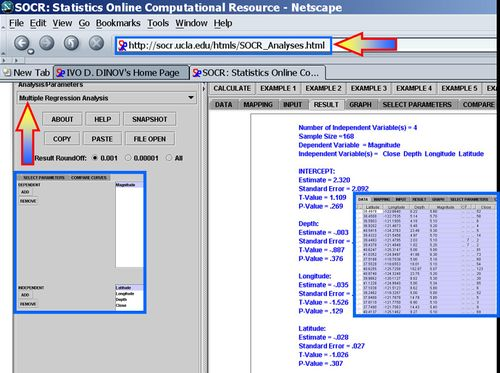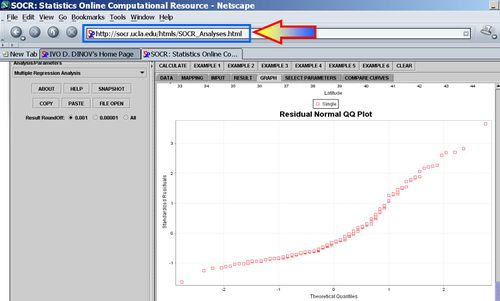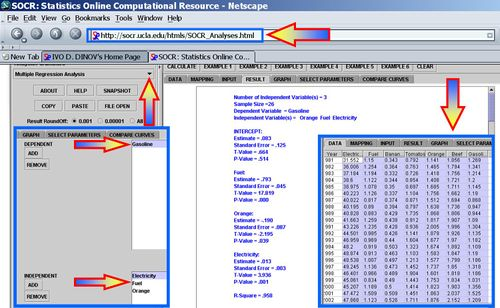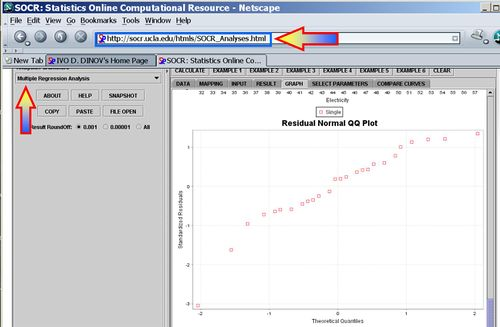# AP Statistics Curriculum 2007 GLM MultLin

## General Advance-Placement (AP) Statistics Curriculum - Multiple Linear Regression

In the previous sections, we saw how to study the relations in bivariate designs. Now we extend that to any finite number of variables (multivariate case).

### Multiple Linear Regression

We are interested in determining the linear regression, as a model, of the relationship between one dependent variable Y and many independent variables Xi, i = 1, ..., p. The multilinear regression model can be written as

$Y = \beta_0 + \beta_1 X_1 + \beta_2 X_2 + \cdots +\beta_p X_p + \varepsilon$, where $$\varepsilon$$ is the error term.

The coefficient $$\beta_0$$ is the intercept ("constant" term) and $$\beta_i$$s are the respective parameters of the p independent variables. There are p+1 parameters to be estimated in the multilinear regression.

• Multilinear vs. non-linear regression: This multilinear regression method is "linear" because the relation of the response (the dependent variable $$Y$$) to the independent variables is assumed to be a linear function of the parameters $$\beta_i$$. Note that multilinear regression is a linear modeling technique not because is that the graph of $$Y = \beta_{0}+\beta x$$ is a straight line nor because $$Y$$ is a linear function of the X variables. But the "linear" terms refers to the fact that $$Y$$ can be considered a linear function of the parameters ( $$\beta_i$$), even though it is not a linear function of $$X$$. Thus, any model like

$Y = \beta_o + \beta_1 x + \beta_2 x^2 + \varepsilon$
is still one of linear regression, that is, linear in $$x$$ and $$x^2$$ respectively, even though the graph on $$x$$ by itself is not a straight line.

### Parameter Estimation in Multilinear Regression

A multilinear regression with p coefficients and the regression intercept β0 and n data points (sample size), with $$n\geq (p+1)$$ allows construction of the following vectors and matrix with associated standard errors:

$\begin{bmatrix} y_{1} \\ y_{2} \\ \vdots \\ y_{n} \end{bmatrix} = \begin{bmatrix} 1 & x_{11} & x_{12} & \dots & x_{1p} \\ 1 & x_{21} & x_{22} & \dots & x_{2p} \\ \vdots & \vdots & \vdots & & \vdots \\ 1 & x_{n1} & x_{n2} & \dots & x_{np} \end{bmatrix} \begin{bmatrix} \beta_0 \\ \beta_1 \\ \vdots \\ \beta_p \end{bmatrix} + \begin{bmatrix} \varepsilon_1\\ \varepsilon_2\\ \vdots\\ \varepsilon_n \end{bmatrix}$

or, in vector-matrix notation

$\ y = \mathbf{X}\cdot\beta + \varepsilon.\,$ Each data point can be given as $$(\vec x_i, y_i)$$, $$i=1,2,\dots,n.$$. For n = p, standard errors of the parameter estimates could not be calculated. For n less than p, parameters could not be calculated.

• Point Estimates: The estimated values of the parameters $$\beta_i$$ are given as

$\widehat{\beta}$$$=(\mathbf{X}^T\mathbf{X})^{-1}\mathbf{X}^T {\vec y}$$

• Residuals: The residuals, representing the difference between the observations and the model's predictions, are required to analyse the regression and are given by:

$\hat\vec\varepsilon = \vec y - \mathbf{X} \hat\beta\,$

The standard deviation, $$\hat \sigma$$ for the model is determined from

${\hat \sigma = \sqrt{ \frac {\hat\vec\varepsilon^T \hat\vec\varepsilon} {n-p-1}} = \sqrt {\frac{{ \vec y^T \vec y - \hat\vec\beta^T \mathbf{X}^T \vec y}}[[:Template:N - p - 1]]} }$

The variance in the errors is Chi-square distributed: $\frac{(n-p-1)\hat\sigma^2}{\sigma^2} \sim \chi_{n-p-1}^2$

• Interval Estimates: The $$100(1-\alpha)%$$ confidence interval for the parameter, $$\beta_i$$, is computed as follows:

${\widehat \beta_i \pm t_{\frac{\alpha }{2},n - p - 1} \hat \sigma \sqrt {(\mathbf{X}^T \mathbf{X})_{ii}^{ - 1} } }$,

where t follows the Student's t-distribution with $$n-p-1$$ degrees of freedom and $$(\mathbf{X}^T \mathbf{X})_{ii}^{ - 1}$$ denotes the value located in the $$i^{th}$$ row and column of the matrix.

The regression sum of squares (or sum of squared residuals) SSR (also commonly called RSS) is given by:

${\mathit{SSR} = \sum {\left( {\hat y_i - \bar y} \right)^2 } = \hat\beta^T \mathbf{X}^T \vec y - \frac{1}{n}\left( { \vec y^T \vec u \vec u^T \vec y} \right)}$,

where $$\bar y = \frac{1}{n} \sum y_i$$ and $$\vec u$$ is an n by 1 unit vector (i.e. each element is 1). Note that the terms $$y^T u$$ and $$u^T y$$ are both equivalent to $$\sum y_i$$, and so the term $$\frac{1}{n} y^T u u^T y$$ is equivalent to $$\frac{1}{n}\left(\sum y_i\right)^2$$.

The error (or explained) sum of squares (ESS) is given by:

${\mathit{ESS} = \sum {\left( {y_i - \hat y_i } \right)^2 } = \vec y^T \vec y - \hat\beta^T \mathbf{X}^T \vec y}.$

The total sum of squares (TSS) is given by

${\mathit{TSS} = \sum {\left( {y_i - \bar y} \right)^2 } = \vec y^T \vec y - \frac{1}{n}\left( { \vec y^T \vec u \vec u^T \vec y} \right) = \mathit{SSR}+ \mathit{ESS}}.$

### Examples

We now demonstrate the use of SOCR Multilinear regression applet to analyze multivariate data.

#### Earthquake Modeling

This is an example where the relation between variables may not be linear or explanatory. In the simple linear regression case, we were able to compute by hand some (simple) examples. Such calculations are much more involved in the multilinear regression situations. Thus we demonstrate multilinear regression only using the SOCR Multiple Regression Analysis Applet.

Use the SOCR California Earthquake dataset to investigate whether Earthquake magnitude (dependent variable) can be predicted by knowing the longitude, latitude, distance and depth of the quake. Clearly, we do not expect these predictors to have a strong effect on the earthquake magnitude, so we expect the coefficient parameters not to be significantly distinct from zero (null hypothesis). SOCR Multilinear regression applet reports this model:

$Magnitude = \beta_o + \beta_1\times Close+ \beta_2\times Depth+ \beta_3\times Longitude+ \beta_4\times Latitude + \varepsilon.$

$Magnitude = 2.320 + 0.001\times Close -0.003\times Depth -0.035\times Longitude -0.028\times Latitude + \varepsilon.$#### Multilinear Regression on Consumer Price Index

Using the SOCR Consumer Price Index Dataset we can explore the relationship between the prices of various products and commodities. For example, regressing Gasoline on the following three predictor prices: Orange Juice, Fuel and Electricity illustrates significant effects of all these variables as significant explanatory prices (at $$\alpha=0.05$$) for the cost of Gasoline between 1981 and 2006.

$Gasoline = 0.083 -0.190\times Orange +0.793\times Fuel +0 .013\times Electricity$